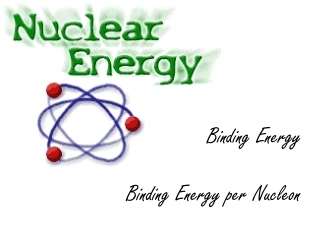Download PresentationBinding Energy Binding Energy per Nucleon

Loading in 2 Seconds...

# Binding Energy Binding Energy per Nucleon

Download Presentation## Binding Energy Binding Energy per Nucleon

- - - - - - - - - - - - - - - - - - - - - - - - - - - E N D - - - - - - - - - - - - - - - - - - - - - - - - - - -
##### Presentation Transcript

1. Binding EnergyBinding Energy per Nucleon

2. Binding Energy The term binding energy is used to indicate the energy that would be required to form an atom from separate protons, electrons and neutrons. The binding energy is therefore the work which would need to be done to separate the atom into its individual particles. N.B. Binding energy is not the energy holding the atom together. So, when an atom is formed, neutrons and protons fall into the nuclear potential well of the nucleus and their potential energy falls also. If we assume zero PE when separated, their PE falls to a negative value. For each isotope, the binding energy per nucleon can be calculated by dividing the energy equivalent of the mass defect by the number of nucleons.

3. Binding Energy Curve Join the correct description with an arrow on the correct place on the graph. If these atoms can be joined together to form heavier atoms, the mass defect increases, so energy has been released If these atoms are split into two nearly equal parts energy is released and again the mass defect increases Any alteration of the nuclear structure which causes a movement towards this point results in released energy. In fact, there is so much of this element in the universe because of its highest mass defect

4. Binding Energy • The previous graph tells us that: • Maximum B.E. per nucleon is at about A = 50 (Fe). In this region the nuclei will be more stable because they possess a greater B.E. per nucleon, which means it requires more energy to lift a nucleon from the potential well of the nucleus (which is at its deepest). • Either side of maximum B.E. per nucleon the nuclei are less stable because they possess less B.E. per nucleon, which means it is easier to separate the nucleus in its constituents nucleons, i.e. the nucleons are not so tightly bound together as at the maximum

5. Binding Energy (continued) • When a big nucleus disintegrates, each nucleon becomes a member of a smaller nucleus, so the B.E. per nucleon increases. This disintegration has created a deeper potential well; the nucleons drop down even further, so their B.E. increases. The loss of PE, i.e. increase of B.E., is released as KE of the product nuclei. Fission occurs when the original nucleus splits into two approximately equal halves. Radioactive disintegration occurs when the original nucleus loses an a, b, or g particle. When these emissions occur the nucleus becomes lighter than before, so that the B.E. per nucleon increases and the nucleus is more stable. • Fusion happens when light nuclei join together. When the product nucleus has A < 50, the B.E. per nucleon is increased by the fusion process and energy is released.